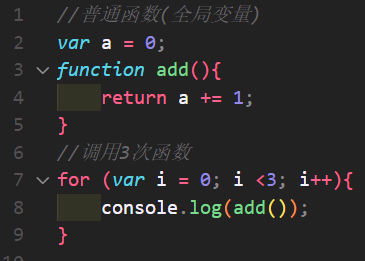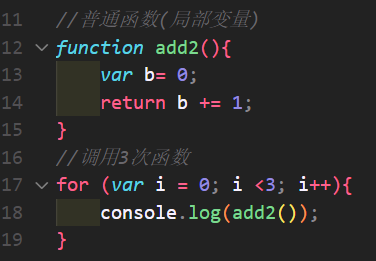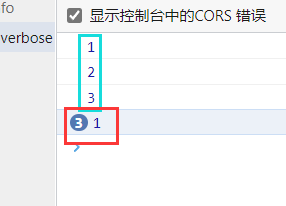### 特殊权限？1
2
3//闭包函数
var b= 0;
return function(){
return b += 1;
}
}

 //普通函数(全局变量)
var a = 0;
return a += 1;
}
//调用3次函数
for (var i = 0; i <3; i++){
}
​
//普通函数(局部变量)
var b= 0;
return b += 1;
}
//调用3次函数
for (var i = 0; i <3; i++){
}
​
//闭包函数
var b= 0;
return function(){
return b += 1;
}
}
//调用请自行琢磨

### 闭包优势

JS规定在一个函数作用域内，程序执行完以后变量就会被销毁，这样可节省内存